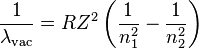# Ionisation Energy of Hydrogen

## Homework Statement

The wavelengths λ of spectral lines produced by the Hydrogen atom are given by the expression:.

Calculate the Energy in eV required to ionise the atom

## Homework Equations

p=h/$$\lambda$$

## The Attempt at a Solution

n2 = 1 and n1 = infinity, therefore $$\lambda$$=1/R.

E = p2/2m = h2/($$\lambda$$^2*2Me)

Subbing in $$\lambda$$=1/R. and rearranging gives:

E = (h2R2)/(2Me)

Why doesn't this give me the right answer?

Last edited:

Redbelly98
Staff Emeritus
Homework Helper

## Homework Equations

p=h/$$\lambda$$

In that equation, λ is the deBroglie wavelength of the electron, and not the wavelength of the photon. So I'm afraid it isn't relevant here.

Instead, what other equation do you have that relates the energy and wavelength of a photon?

In that equation, λ is the deBroglie wavelength of the electron, and not the wavelength of the photon. So I'm afraid it isn't relevant here.

Instead, what other equation do you have that relates the energy and wavelength of a photon?

So it's just as simple as using E = hc/lamda = hcR?

Redbelly98
Staff Emeritus
Homework Helper
Yes.ah i see what's going on now - the equation gives the wavelength of the emitted photon not the wavelength of the electron? Thanks.

Redbelly98
Staff Emeritus